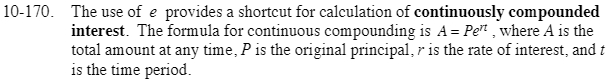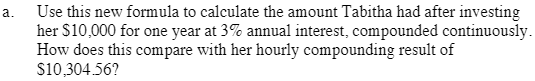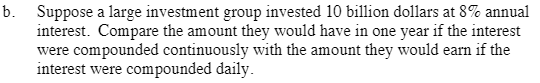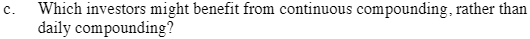### Home > CCA2 > Chapter Ch10 > Lesson 10.3.2 > Problem10-170

10-170.
1. The use of e provides a shortcut for calculation of continuously compounded interest. The formula for continuous compounding is A = Pert, where A is the total amount at any time, P is the original principal, r is the rate of interest, and t is the time period. Homework Help ✎

1. Use this new formula to calculate the amount Tabitha had after investing her $10,000 for one year at 3% annual interest, compounded continuously. How does this compare with her hourly compounding result of$10,304.56?

2. Suppose a large investment group invested 10 billion dollars at 8% annual interest. Compare the amount they would have in one year if the interest were compounded continuously with the amount they would earn if the interest were compounded daily.

3. Which investors might benefit from continuous compounding, rather than daily compounding?A = 10,000e(0.03)(1)$\text{For the interest compounded daily, use }A=10,000,000,000\left( 1+\frac{0.08}{365} \right)^{365}.$